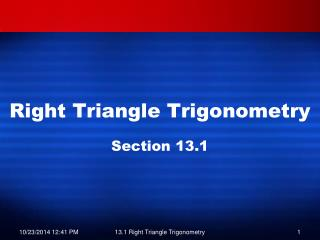DownloadDownload PresentationRight Triangle Trigonometry

# Right Triangle Trigonometry

Download Presentation## Right Triangle Trigonometry

- - - - - - - - - - - - - - - - - - - - - - - - - - - E N D - - - - - - - - - - - - - - - - - - - - - - - - - - -
##### Presentation Transcript

1. Right Triangle Trigonometry Section 13.1 13.1 Right Triangle Trigonometry

2. Definitions • Trigonometry • Comes from Greek word – Trigonon, which means 3 angles • “Metry” means measure in Greek • Trigonometry Ratios • Sine, Cosine, Tangent, Secant, Cosecant, Cotangent • Types of angles • Acute: Less than 90° • Equilateral: 90° • Obtuse: More than 90° but less than 180° 13.1 Right Triangle Trigonometry

3. Right Triangles • Consider a right triangle, one of whose acute angles is ө • The three sides of a triangle are hypotenuse, opposite, and adjacent side of ө • To determine what is the opposite side, look at өand extend the line to determine the opposite hypotenuse opposite adjacent 13.1 Right Triangle Trigonometry

4. Right Triangles SOHCAHTOA Sine ө= Cosine ө = Tangent ө = SIN COS TAN Reciprocals of SOHCAHTOA Cosecant ө = Secant ө = Cotangent ө= CSC SEC COT 13.1 Right Triangle Trigonometry

5. Right Triangles Relationships of Trigonometric Ratios Sine ө = Cosecant ө = SIN CSC Cosine ө = Secant ө = COS SEC Tangent ө = Cotangent ө = TAN COT 13.1 Right Triangle Trigonometry

6. Steps in Determining Triangles • Solve for x, using Pythagorean Theorem • Determine the hypotenuse and the opposite by identifying ө • Use Trigonometry Functions to find what’s needed 13.1 Right Triangle Trigonometry

7. Example 1 Step 1: Find x Find x and determine all trig functions of ө 13 12 x Use the Pythagorean Theorem to find the length of the adjacent side… a2 + 122=132 a2=25 a = 5 13.1 Right Triangle Trigonometry

8. Example 1 Step 2: Determine the hypotenuse and the opposite by identifying ө Find x and determine all trig functions of ө 13 12 x adj = 5 opp = 12 hyp = 13 13.1 Right Triangle Trigonometry

9. 13 12 5 Example 1 Step 3: Use Trigonometry Functions to find what’s needed Find x and determine all trig functions of ө • Sine ө= Cosine ө = Tangent ө = • SIN COS TAN • Cosecant ө = Secant ө = Cotangent ө= • CSC SEC COT 13.1 Right Triangle Trigonometry

10. 13 12 5 Example 1 Find x and determine all trig functions of ө Step 3: Use Trigonometry Functions to find what’s needed • Sine ө= Cosine ө = Tangent ө = • SIN COS TAN • Cosecant ө = Secant ө = Cotangent ө= • CSC SEC COT 13.1 Right Triangle Trigonometry

11. Your Turn Determine all trig functions of ө 2 1 13.1 Right Triangle Trigonometry

12. 2 1 Your Turn Determine all trig functions of ө • Sine ө= Cosine ө = Tangent ө = • SIN COS TAN • Cosecant ө = Secant ө = Cotangent ө= • CSC SEC COT Can we have radicals in the denominators? Actually, with trig ratios, it is accepted in the subject area. But it is necessary to simplify radicals 13.1 Right Triangle Trigonometry

13. Example 2 What is given? • Hypotenuse: 74 • Opposite of 30°: x • Adjacent: Unknown Which of the six trig ratios is best fit for this triangle? (there can be more than one answer) Solve for x. 13.1 Right Triangle Trigonometry

14. Example 2 Which of the six trig ratios is best fit for this triangle? (there can be more than one answer) Solve for x. Must change the answer to DEGREE mode and not RADIAN mode in calculator 13.1 Right Triangle Trigonometry

15. Example a Find the value of sine, cosine and tangent functions

16. Example 3 In a waterskiing competition, a jump ramp has the measurements shown. To the nearest foot, what is the height h above water that a skier leaves the ramp? Substitute 15.1° for θ, h for opposite, and 19 for hypotenuse. Multiply both sides by 19. Use a calculator to simplify. The height above the water is about 5 ft. 13.1 Right Triangle Trigonometry

17. Your Turn Solve for h. Round to 4 decimal places 13.1 Right Triangle Trigonometry

18. Your Turn Solve for the rest of missing sides of triangle ABC, given that A = 35° and c = 15.67. Round to 4 decimal places 13.1 Right Triangle Trigonometry

19. Example b Find the value of x 10

20. Example c Find the value of x. 12

21. Example d Find the value of x. 8

22. Angle of Elevation vs. Depression • Angle of Elevation is a measurement above the horizontal line • Angle of Depressionis a measurement below the horizontal line Angle of Elevation Angle of Depression 6.2 Trig Applications

23. Example 4 A flagpole casts a 60-foot shadow when the angle of elevation of the sun is 35°. Find the height of the flagpole. 35° ---- 60 Feet ---- 6.2 Trig Applications

24. Example 4 A flagpole casts a 60-foot shadow when the angle of elevation of the sun is 35°. Find the height of the flagpole. 35° ---- 60 Feet ---- 6.2 Trig Applications

25. Example 5 Find the distance of a boat from a lighthouse if the lighthouse is 100 meters tall, and the angle of depression is 6°. 6.2 Trig Applications

26. Your Turn A man who is 2 m tall stands on horizontal ground 30 m from a tree. The angle of elevation of the top of the tree from his eyes is 28˚. Estimate the height of the tree. 6.2 Trig Applications

27. Example e Solve 45 ft

28. Assignment Pg 933 3-25 odd 13.1 Right Triangle Trigonometry